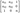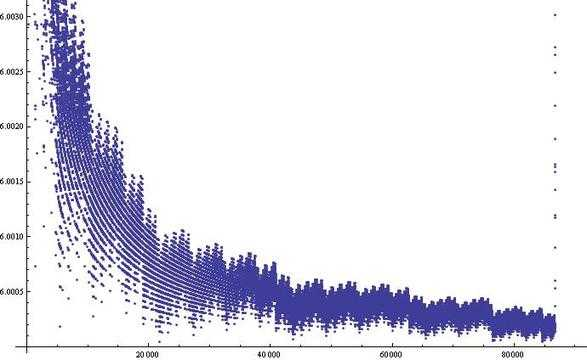## 三平方和

Sat, 18th January 2020Edit on Githubsquareunimodular

2011年3月KeyTo9_Fans提问:

$A_1=7$
$A_2=15$
$A_3=23$
$A_4=28$
$A_5=31$
……

(1)$A_n>6n$
(2)$\lim\limits_{n\to\infty}\frac{A_n}n=6$

# 初步分析

mathe根据A004215中信息发现这个数列就是$4^a(8k+7)$, 但是这个结论比较困以证明。

8k+7型的有$[\frac{n+1}8]$
4(8k+7)型的有$[\frac{n+4}{32}]$
..
$4^h(8k+7)$型的有$[\frac{n+4^h}{2^{2h+3}}]$

zgg__给出了数列$\frac{A_n}n$图像:KeyTo9_Fans也指出，证明(8k+7)的正整数不能表示为3个平方数之和很容易

$0^2 \mod 8=0$
$1^2 \mod 8=1$
$2^2 \mod 8=4$
$3^2 \mod 8=1$
$4^2 \mod 8=0$
$5^2 \mod 8=1$
$6^2 \mod 8=4$
$7^2 \mod 8=1$

$0=0+0+0$
$1=1+0+0$
$2=1+1+0$
$3=1+1+1$
$4=4+0+0$
$5=4+1+0$
$6=4+1+1$
$7$不能用$3$$0$$1$$4$来表示。

1. $4(8k+7)$$16(8k+7)$、……的正整数也不能表示成$3$个平方数之和。
2. 其余的正整数可以表示成$3$个平方数之和。

mathe指出，$4^a(8b+7)$不能表示成三平方数和也简单。由于是偶数，那么三个平方数必然全部偶数或两奇一偶。

zgg__指出，根据A004215记载，高斯最早解决了三平方和问题。

# 幺模矩阵

mathe从网络上找到了证明过程, 其中主要使用了一种叫做幺模矩阵(Unimodular Matrix)的数学工具。 幺模矩阵就是那些所有元素都是整数，而且行列式是1或-1的矩阵。

$Ax=b=\begin{bmatrix}0\\0\\ \vdots\\0\\1\\0\\ \vdots\\0\end{bmatrix}$

# 幺模等价关系

$g(x)=x^{\prime}T^{\prime}ATx=x^{\prime}Bx$,对于任意$a=b^{\prime}Ab$,取$c=T^{-1}b$,那么$a=c^{\prime}Bc$,反之亦然。所以两个函数值域相等。

# 证明简介

$x=\begin{pmatrix}0\\0\\1\end{pmatrix}$

# 三立方和

$42=(-80538738812075974)^3 + 80435758145817515^3 + 12602123297335631^3$

i) 有无穷种将1表示为三立方和的方案
$(1\pm 9m^3)^3 + (9m^4)^3 + (-9m^4 \mp 3m)^3 = 1$
ii) Ramanujan给出了
$(3n^2+5nm-5m^2)^3+(4n^2-4nm+6m^2)^3+(5n^2-5nm-3m^2)^3=(6n^2-4nm+4m^2)^3$

iii) 当$n\equiv 4(\mod 9)$$n\equiv 5(\mod 9)$时，$x^3+y^3+z^3=n$无整数解
iv) 我们可以猜测除了iii)中情况，所有其它n可以表示为三个立方数之和， 但是这个问题还无人能够证明。

Github